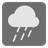Flood Area
Simple
English RU

You are given a cross-section diagram (a string). It represents slopes and flatlands by '/', '\' and '_' respectively. For example, the region of the following picture is shown by a string "/\\///\_/\/\\\\/_/\\///__\\\_\\/_\/_/".

Assume that the rain is falling endlessly and the water overflowing from the region is falling into the sea from both sides. In the following example the rain will create floods in 4, 2, 1, 19 and 9 areas respectively.

You should return a list (or an iterable) of the numbers of floods from the left side of the cross-section diagram. If there aren’t any floods, return [] (or an empty iterable).Example:

```list(flood_area(r'\\//')) == 
list(flood_area(r'/\\///\_/\/\\\\/_/\\///__\\\_\\/_\/_/')) == [4, 2, 1, 19, 9]
list(flood_area(r'_/_\_')) == []
```

Input: A cross-section diagram (a string).

Output: The numbers of floods (a list/iterable of integers).

Precondition:

• 1 ≤ len(diagram) ≤ 500

The mission was taken from AIZU ONLINE JUDGE(ALDS1_3_D: Areas on the Cross-Section Diagram).